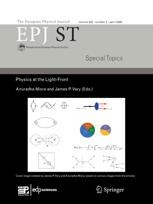# Infinite number of quantum particles gives clues to big-picture behaviour at large scale

Scientists gain a deeper understanding of phenomena at macroscopic scale by simulating the consequences of having an infinite number of physical phenomena at quantum scale

New York | Heidelberg, 9 April 2019In quantum mechanics, the Heisenberg uncertainty principle prevents an external observer from measuring both the position and speed (referred to as momentum) of a particle at the same time. They can only know with a high degree of certainty either one or the other - unlike what happens at large scales where both are known. To identify a given particle’s characteristics, physicists introduced the notion of quasi-distribution of position and momentum. This approach was an attempt to reconcile quantum-scale interpretation of what is happening in particles with the standard approach used to understand motion at normal scale, a field dubbed classical mechanics.

In a new study published in EPJ Special Topics, Dr J.S. Ben-Benjamin and colleagues from Texas A&M University, USA, reverse this approach; starting with quantum mechanical rules, they explore how to derive an infinite number of quasi-distributions, to emulate the classical mechanics approach. This approach is also applicable to a number of other variables found in quantum-scale particles, including particle spin.

For example, such quasi-distributions of position and momentum can be used to calculate the quantum version of the characteristics of a gas, referred to as the second virial coefficient, and extend it to derive an infinite number of these quasi-distributions, so as to check whether it matches the traditional expression of this physical entity as a joint distribution of position and momentum in classical mechanics.

This approach is so robust that it can be used to replace quasi-distributions of position and momentum with time and frequency distributions. This, the authors note, works for both well-determined scenarios where time and frequency quasi-distributions are known, and for random cases where the average of time and average of frequency are used instead.

References:
From von Neumann to Wigner and beyond.J. S. Ben-Benjamin, L. Cohen and M. O. Scully (2019), Eur. Phys. J. Spec. Top. (2019) 227: 2171. DOI 10.1140/epjst/e2018-800063-2

Further Information